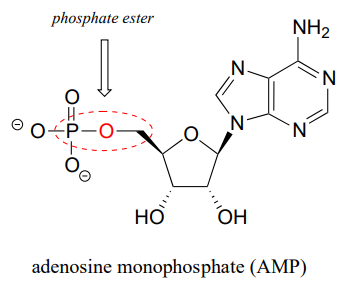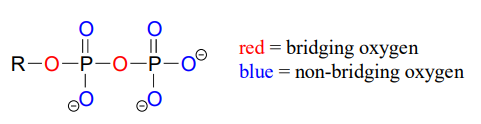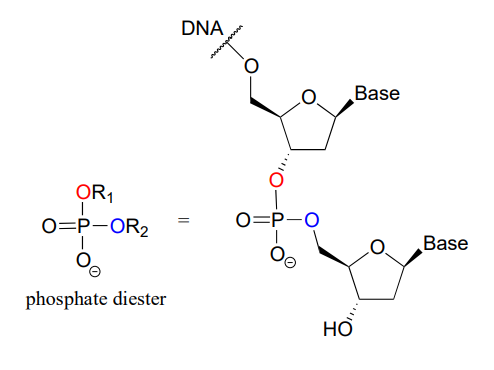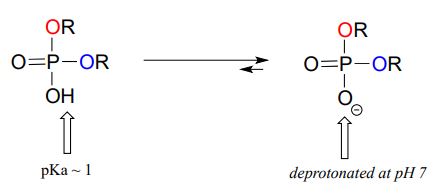# 9.2: Overview of Phosphate Groups

$$\newcommand{\vecs}{\overset { \rightharpoonup} {\mathbf{#1}} }$$ $$\newcommand{\vecd}{\overset{-\!-\!\rightharpoonup}{\vphantom{a}\smash {#1}}}$$$$\newcommand{\id}{\mathrm{id}}$$ $$\newcommand{\Span}{\mathrm{span}}$$ $$\newcommand{\kernel}{\mathrm{null}\,}$$ $$\newcommand{\range}{\mathrm{range}\,}$$ $$\newcommand{\RealPart}{\mathrm{Re}}$$ $$\newcommand{\ImaginaryPart}{\mathrm{Im}}$$ $$\newcommand{\Argument}{\mathrm{Arg}}$$ $$\newcommand{\norm}{\| #1 \|}$$ $$\newcommand{\inner}{\langle #1, #2 \rangle}$$ $$\newcommand{\Span}{\mathrm{span}}$$ $$\newcommand{\id}{\mathrm{id}}$$ $$\newcommand{\Span}{\mathrm{span}}$$ $$\newcommand{\kernel}{\mathrm{null}\,}$$ $$\newcommand{\range}{\mathrm{range}\,}$$ $$\newcommand{\RealPart}{\mathrm{Re}}$$ $$\newcommand{\ImaginaryPart}{\mathrm{Im}}$$ $$\newcommand{\Argument}{\mathrm{Arg}}$$ $$\newcommand{\norm}{\| #1 \|}$$ $$\newcommand{\inner}{\langle #1, #2 \rangle}$$ $$\newcommand{\Span}{\mathrm{span}}$$$$\newcommand{\AA}{\unicode[.8,0]{x212B}}$$

Phosphate is everywhere in biochemistry. As we were reminded in the introduction to this chapter, our DNA is linked by phosphate:The function of many proteins is regulated - switched on and off - by enzymes which attach or remove a phosphate group from the side chains of serine, threonine, or tyrosine residues.Countless diseases are caused by defects in phosphate transferring enzymes. As just one example, achondroplasia, a common cause of dwarfism, is caused by a defect in an enzyme whose function is to transfer a phosphate to a tyrosine residue in a growth-related signaling protein.

Finally, phosphates are excellent leaving groups in biological organic reactions, as we will see many times throughout the remainder of this book.

Clearly, an understanding of phosphate chemistry is central to the study of biological organic chemistry. We'll begin with an overview of terms used when talking about phosphates.

## Terms and abbreviations

The fully deprotonated conjugate base of phosphoric acid is called a phosphate ion, or inorganic phosphate (often abbreviated '$$P_i$$'). When two phosphate groups are linked to each other, the linkage itself is referred to as a 'phosphate anhydride', and the compound is called 'inorganic pyrophosphate' (often abbreviated $$PP_i$$).The chemical linkage between phosphate and a carbon atom is a phosphate ester. Adenosine monophosphate (AMP) has a single phosphate ester linkage.Oxygen atoms in phosphate groups are referred to either as 'bridging' or 'non-bridging', depending on their position. An organic diphosphate has two bridging oxygens (one in the phosphate ester linkage and one in the phosphate anhydride linkage) and five non-bridging oxygens:A single phosphate is linked to two organic groups is called phosphate diester. The backbone of DNA is linked by phosphate diesters.Organic phosphates are often abbreviated using '$$OP$$' and '$$OPP$$' for mono- and diphosphates, respectively. For example, glucose-6-phosphate and isopentenyl diphosphate are often depicted as shown below. Notice that the 'P' abbreviation includes the associated oxygen atoms and negative charges.##### Exercise 9.2.1

Consider the biological compounds below, some of which are shown with abbreviated structures:1. For each compound, specify the number of bridging and non-bridging oxygens in the phosphate group.

## Acid constants and protonation states

Phosphoric acid is triprotic, meaning that it has three acidic protons available to donate, with $$pK_a$$ values of 1.0, 6.5, and 13.0, respectively. (da Silva and Williams)These acid constant values, along with the Henderson-Hasselbalch equation (section 7.2) tell us that, at the physiological $$pH$$ of approximately 7, somewhat more than half of the phosphate species will be in the $$HPO_4^{-2}$$ state, and slightly less than half will be in the $$H_2PO_4^{-1}$$ state, meaning that the average net charge is between -1.5 and -2.0.

Phosphate diesters have a $$pK_a$$ of about 1, meaning that they carry a full negative charge at physiological $$pH$$.Organic monophosphates, diphosphates, and triphosphates all have net negative charges and are partially protonated at physiological $$pH$$, but by convention are usually drawn in the fully deprotonated state.

##### Exercise 9.2.2

Explain why the second $$pK_a$$ of phosphoric acid is so much higher than the first $$pK_a$$.

##### Exercise 9.2.3

What is the approximate net charge of inorganic phosphate in a solution buffered to $$pH 1$$?

Recall from section 8.4 that good leaving groups in organic reactions are, as a rule, weak bases. In laboratory organic reactions, leaving groups are often halides or toluenesulfonates (section 8.4), both of which are weak bases. In biological organic reactions, phosphates are very common leaving groups. These could be inorganic phosphate, inorganic pyrophosphate, or organic monophosphates, all of which are weakly basic, especially when coordinated to metal cations such as $$Mg^{+2}$$ in the active site of an enzyme. We will see many examples of phosphate leave groups in this and subsequent chapters.

## Bonding in phosphates

Looking at the location of phosphorus on the periodic table, you might expect it to bond and react in a fashion similar to nitrogen, which is located just above it in the same column. Indeed, phosphines - phosphorus analogs of amines - are commonly used in the organic laboratory.However it is in the form of phosphate, rather than phosphine, that phosphorus plays its main role in biology.

The four oxygen substituents in phosphate groups are arranged about the central phosphorus atom with tetrahedral geometry, however there are a total of five bonds to phosphorus - four s bonds and one delocalized $$\pi$$ bond.Phosphorus can break the 'octet rule' because it is on the third row of the periodic table, and thus has $$d$$ orbitals available for bonding. The minus 3 charge on a fully deprotonated phosphate ion is spread evenly over the four oxygen atoms, and each phosphorus-oxygen bond can be considered to have 25% double bond character: in other words, the bond order is 1.25.

Recall from section 2.1 the hybrid bonding picture for the tetrahedral nitrogen in an amine group: a single $$2s$$ and three $$2p$$ orbitals combine to form four $$sp^3$$ hybrid orbitals, three of which form s bonds and one of which holds a lone pair of electrons.In the hybrid orbital picture for phosphate ion, a single $$3s$$ and three $$3p$$ orbitals also combine to form four $$sp^3$$ hybrid orbitals with tetrahedral geometry. In contrast to an amine, however, four of the five valance electrons on phosphorus occupy $$sp^3$$ orbitals, and the fifth occupies an unhybridized $$3d$$ orbital.This orbital arrangement allows for four s bonds with tetrahedral geometry in addition to a fifth, delocalized $$p$$ bond formed by $$p$$ overlap between the half-filled $$3d$$ orbital on phosphorus and $$2p$$ orbitals on the oxygen atoms.

In phosphate esters, diesters, and anhydrides the π bonding is delocalized primarily over the non-bridging bonds, while the bridging bonds have mainly single-bond character. In a phosphate diester, for example, the two non-bridging oxygens share a -1 charge, as illustrated by the two major resonance contributors below. The bonding order for the bridging $$P-O$$ bonds in a phosphate diester group is about 1, and for the non-bridging $$P-O$$ bonds about 1.5. In the resonance contributors in which the bridging oxygens are shown as double bonds (to the right in the figure below), there is an additional separation of charge - thus these contributors are minor and make a relatively unimportant contribution to the overall bonding picture.##### Exercise 9.2.4

Draw all of the resonance structures showing the delocalization of charge on a (fully deprotonated) organic monophosphate. If a 'bond order' of 1.0 is a single bond, and a bond order of 2.0 is a double bond, what is the approximate bond order of bridging and non-bridging $$P-O$$ bonds?

Throughout this book, phosphate groups will often be drawn without attempting to show tetrahedral geometry, and π bonds and negative charges will usually be shown localized to a single oxygen. This is done for the sake of simplification - however it is important always to remember that the phosphate group is really tetrahedral, the negative charges are delocalized over the non-bridging oxygens, and that there is some degree of protonation at physiological $$pH$$ (with the exception of the phosphate diester group).

This page titled 9.2: Overview of Phosphate Groups is shared under a CC BY-NC-SA 4.0 license and was authored, remixed, and/or curated by Tim Soderberg via source content that was edited to the style and standards of the LibreTexts platform; a detailed edit history is available upon request.